##### Quantum Physics For Dummies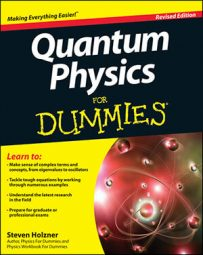A multi-electron atom is the most common multi-particle system that quantum physics considers. You can apply a Hamiltonian wave function to a neutral, multi-electron atom, as shown in the following figure. Here, R is the coordinate of the nucleus (relative to the center of mass), r1 is the coordinate of the first electron (relative to the center of mass), r2 the coordinate of the second electron, and so on.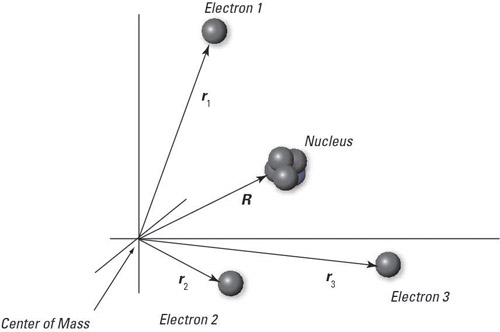A multi-electron atom.

If you have Z electrons, the wave function looks like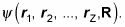And the kinetic energy of the electrons and the nucleus looks like this: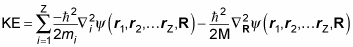And the potential energy of the system looks like this: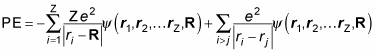So adding the two preceding equations, here’s what you get for the total energy (E = KE + PE) of a multi-particle atom: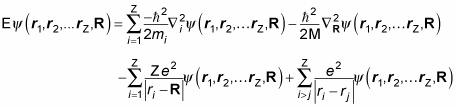Okay, now that looks like a proper mess. Want to win the Nobel prize in physics? Just come up with the general solution to the preceding equation. As is always the case when you have a multi-particle system in which the particles interact with each other, you can’t split this equation into a system of N independent equations.

In cases where the N particles of a multi-particle system don’t interact with each other, where you can disconnect the Schrödinger equation into a set of N independent equations, solutions may be possible. But when the particles interact and the Schrödinger equation depends on those interactions, you can’t solve that equation for any significant number of particles.

However, that doesn’t mean all is lost by any means. You can still say plenty about equations like this one if you’re clever — and it all starts with an examination of the symmetry of the situation.